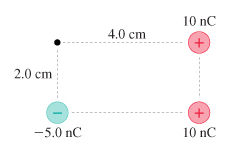# Problem: What are the strength and direction of the electric field at the position indicated by the dot in figure (Figure 1)A) Give your answer in terms of the horizontal and vertical components. Take the positive directions to be up and to the right.B) Give your answer as a magnitude and angle measured from the positive x-axis.

🤓 Based on our data, we think this question is relevant for Professor Haseler's class at GSU.

###### FREE Expert Solution

Electric field:

$\overline{){\mathbf{E}}{\mathbf{=}}\frac{\mathbf{k}\mathbf{q}}{{\mathbf{r}}^{\mathbf{2}}}}$

(A)E1 = kq/r2 = (9.0 × 109)(-5.0 × 10-9)/(0.022) = -112500 N/C j

E2 = kq/r2 = (9.0 × 109)(10.0 × 10-9)/(0.042) = -56250 N/C i###### Problem DetailsWhat are the strength and direction of the electric field at the position indicated by the dot in figure (Figure 1)

A) Give your answer in terms of the horizontal and vertical components. Take the positive directions to be up and to the right.

B) Give your answer as a magnitude and angle measured from the positive x-axis.